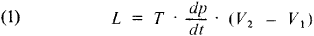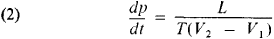# Clausius-Clapeyron Equation

## Clausius-Clapeyron equation

[klȯz·ē·əs kla·pā‚rōn i‚kwā·zhən]
(thermodynamics)
An equation governing phase transitions of a substance, dp/dT = Δ H /(T Δ V), in which p is the pressure, T is the temperature at which the phase transition occurs, Δ H is the change in heat content (enthalpy), and Δ V is the change in volume during the transition. Also known as Clapeyron-Clausius equation; Clapeyron equation.
McGraw-Hill Dictionary of Scientific & Technical Terms, 6E, Copyright © 2003 by The McGraw-Hill Companies, Inc.
The following article is from The Great Soviet Encyclopedia (1979). It might be outdated or ideologically biased.

## Clausius-Clapeyron Equation

a thermodynamic equation relating to the transition processes of a substance from one phase to another (such as vaporization, fusion, sublimation, polymorphic transformation). According to the Clausius-Clapeyron equation, the heat of a phase transition (for example, heat of vaporization, heat of fusion) under equilibrium conditions is defined by the equationwhere T is the temperature of transition (isothermal process), dp/dT is the value of the derivative of pressure with respect to temperature at a given temperature of the transition, and (V2V1) is the change in the volume of the substance during its transition from the first phase to the second.

The equation was initally derived in 1834 by B. P. E. Clapeyron from analysis of the Carnot cycle for the condensation of steam in thermal equilibrium with the liquid. R. Clausius refined the equation in 1850 and extended it to other phase transitions. The Clausius-Clapeyron equation is applicable to any phase transition accompanied by either absorption or evolution of heat (the phase transition of the first order) and is a direct result of the conditions of phase equilibrium from which it is derived.

The Clausius-Clapeyron equation may be used for calculating any of the quantities entering into the equation if the remaining quantities are known. In particular, the equation is used for calculating the heats of evaporation, which are difficult to determine experimentally.

The Clausius-Clapeyron equation may be formulated in terms of the derivatives dp/dT or dT/dp:For evaporation and sublimation processes, dp/dT expresses the change in the saturation vapor pressure p with the temperature T, whereas for the processes of fusion and polymorphic transformation, dT/dp determines the change in the transition temperature with the pressure. In other words, the Clausius-Clapeyron equation is a differential equation of the curve of phase equilibrium in terms of the variables p and T.

In order to solve the Clausius-Clapeyron equation it is necessary to know how the quantities L, V1, and V2 change with temperature and pressure, which is a difficult task. This relationship is usually determined empirically and the Clausius-Clapeyron equation is solved numerically.

The Clausius-Clapeyron equation may be applied to pure substances and to solutions and the separate components of solutions. In the latter cases, the Clausius-Clapeyron equation relates the partial pressure of unsaturated vapor of a given component to its partial heat of vaporization.

### REFERENCE

Kurs fizicheskoi khimii, 2nd ed., vol. 1. Edited by la. I. Gerasimov. Moscow, 1969.

IU. I. POLIAKOV

References in periodicals archive ?
Using the Clausius-Clapeyron equation, the heat of desorption is expressed as:
The mechanical system has a behavior predicted by Boltzmann statistics and therefore also illustrates the essential physics of the Clausius-Clapeyron equation. The mere existence of a potential energy well in a system of agitated particles is sufficient to guarantee that the equilibrium "vapor" density increase with "temperature," a measure of the energy imposed on the particles comprising the system.
The actual heat released by phase change in the atmosphere depends upon many factors, such as the amount of water vapor present, the presence and distribution of condensation nuclei, degree of supercooling, etc., assuming of course that the Clausius-Clapeyron equation is satisfied.
These results indicate that, to within less than half a percentage point (mean error 0.33%) and about 1.15[degrees]C (mean bias), the Clausius-Clapeyron equation can be used to estimate the boiling point temperature of water in pressures typical of Earth's lower atmosphere, even when using a rough estimate of the value of the latent heat of vaporization.
Differential enthalpies were calculated according to Clausius-Clapeyron equation (Clegg, 1978) and tissue affinity for strongly bound water was expressed as average differential enthalpy for moisture content lower than 0.10 g [H.sub.2]O/g dry weight.
In the Clausius-Clapeyron equation, [q.sub.st] was determined from the slopes of the curves of the chart ln([a.sub.w]) x (1/[T.sub.abs]) for the diverse equilibrium moisture contents on a dry basis from the mathematical model that best fit the experimental data, as described in Eqs.
Then by using the Clausius-Clapeyron equation, the vapour pressure corresponding to this temperature is calculated.
The Clausius-Clapeyron equation was used to calculate isosteric heat ( H ) of adsorption.
The isosteric heats of adsorption as a function of the surface coverage can be calculated from isotherms at different temperatures by using Clausius-Clapeyron equation (1) 21,22]:
In contrary to the temperature dependence of diffusion described generally by Clausius-Clapeyron equation (16), the diffusion coefficients for [SO.sub.3] diffusing into SPS grew with increasing temperature mostly because of the further chemical reaction of [SO.sub.3] with PS.
To calculate the isosteric heat (QST) a linear regression of data was run between the natural logarithm of water activity and the reciprocal of temperature at different equilibrium moisture content, and then the Clausius-Clapeyron equation (equation 8) was applied to it.

Site: Follow: Share:
Open / Close